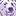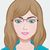# QlikView App Dev

Discussion Board for collaboration related to QlikView App Development.

Announcements
Join us for a live Q&A! September 21, 10 AM ET - Onboarding Fast in Qlik Sense SaaS! REGISTER
cancel
Showing results for
Did you mean:Contributor III

## Input dash with text when variable is null

Hi Everyone,

I'm currently working on a dashboard where I want to input a dash ( - ) when a value is null. Usually this is a default for Qlikview, but within my expression I combine the value with a label as pictured below.The end goal is to have ' - days' instead of just days appear. I also want to avoid placing a zero in front of the days when a value is null because it can be misleading to end users because zero days is different than the null value.

Currently, I am using the following expression to calculate the numbers within the pivot chart. There is a toggle for the pivot chart to show different values, hence the combined if statement.

=if(ShowID_US1='d',Num(Sum({<Year={'Previous Year'}>}TotalDays)/Sum({<Year={'Previous Year'}>}TotalUD),'##.0') & ' days',
if(ShowID_US1='h',Num(Sum({<Year={'Previous Year'}>}TotalDays)/Sum({<Year={'Previous Year'}>}TotalUD),'#,##0') & ' hours'))

I appreciate any help! Thank you!

Labels (2)

• ### QLIKVIEW chart expressions

1 Solution

Accepted SolutionsPartner

Hi,

maybe you could try something like this

=if(ShowID_US1='d',
If(Len(Num(Sum({<Year={'Previous Year'}>}TotalDays)/Sum({<Year={'Previous Year'}>}TotalUD),'##.0'))=0,'-',
Num(Sum({<Year={'Previous Year'}>}TotalDays)/Sum({<Year={'Previous Year'}>}TotalUD),'##.0')) & ' days',
if(ShowID_US1='h',
If(Len(Num(Sum({<Year={'Previous Year'}>}TotalDays)/Sum({<Year={'Previous Year'}>}TotalUD),'#,##0'))=0,'-',
Num(Sum({<Year={'Previous Year'}>}TotalDays)/Sum({<Year={'Previous Year'}>}TotalUD),'#,##0')) & ' hours'))

3 RepliesCreator II

Hi,

Check mark the Suppress zero values i presentation tab. May it helpsContributor III
Author

Although the value is null, I don't want to suppress the value, instead I want to make it a dash. The problem comes in with combining the numeric and string characters within the expression that I am unsure how to deal with.Partner

Hi,

maybe you could try something like this

=if(ShowID_US1='d',
If(Len(Num(Sum({<Year={'Previous Year'}>}TotalDays)/Sum({<Year={'Previous Year'}>}TotalUD),'##.0'))=0,'-',
Num(Sum({<Year={'Previous Year'}>}TotalDays)/Sum({<Year={'Previous Year'}>}TotalUD),'##.0')) & ' days',
if(ShowID_US1='h',
If(Len(Num(Sum({<Year={'Previous Year'}>}TotalDays)/Sum({<Year={'Previous Year'}>}TotalUD),'#,##0'))=0,'-',
Num(Sum({<Year={'Previous Year'}>}TotalDays)/Sum({<Year={'Previous Year'}>}TotalUD),'#,##0')) & ' hours'))Community Browser